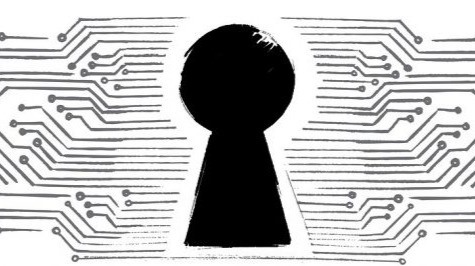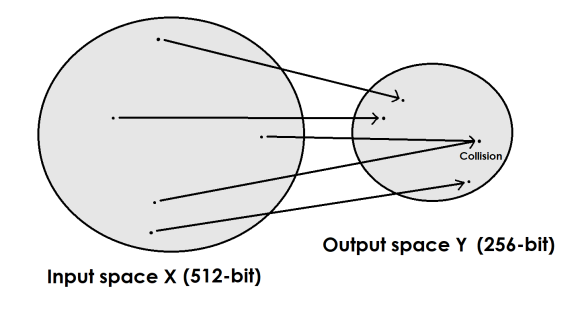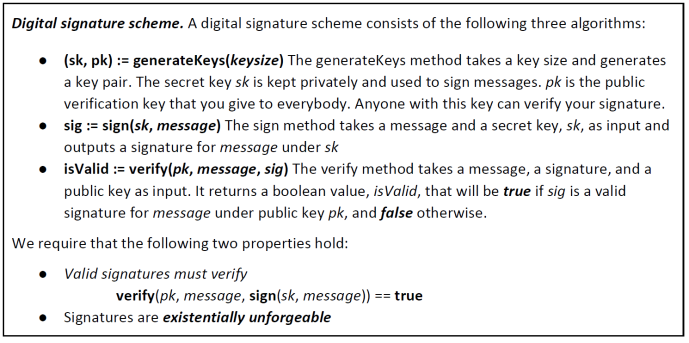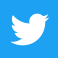6 - Understanding cryptographic hash functions# 6 - Understanding cryptographic hash functions

By CryptoGameTheory | Crypto4Friends | 28 Jul 2020

Before understanding how the Blockchain works we have to analyze its main components. The first cryptographic primitive that we'll need to understand is a cryptographic hash function. A hash function is a mathematical hashing algorithm that produces a digital fingerprint or "hash".
It allows us to take any input of data - a string of arbitrary size, producing an output of fixed size, that in the case of the Bitcoin's Blockchain is 256-bit, or 2^256 bits. When you hash data, such for instance the sentence

Hello! Thanks for tipping!

the resulting hash would be something like:

3774d10b7fe6d1540968ca1f8bb8da10d9a9d0951aa758f3b763cc487e7d8861

The hash function, to be useful for the Blockchain, has to be efficiently computable and cryptographically secure. In the first case it means that, given the input string, you can figure out what the output of the hash function is, in a reasonable amount of time. In the second case, to achieve security, we're going to require that the hash function has the following three additional properties:

• Collision-resistance: "A hash function H is said to be collision resistant if it is infeasible to find two values, x and y, such that x is not equal to y, yet H(x)=H(y) ."If this condition is respected, it means that it is infeasible to find the same output by hashing two different files. In other words, nobody can find a collision. Notice that we said infeasible, not impossible. Indeed, it is proven that collisions exist, but detect them will take a very long time. This is because the input space is much bigger than the output space and because the number space of 256-bits is an unimaginable number.  To appreciate its size we can decompose it in smaller pieces, that is 2^32 2^32 2^32 2^32, where 2^32 is roughly 4 billion.

Now, suppose that we want to find a collision and suppose that we have a really good GPU that can calculate 1 billion Hashes per second (H/s). With four GPUs we reach the first 4 billion hashes. Then, suppose to pick 4 billion of these calculators to form a supercomputer, much powerful than all the Google's server combined. You could give one supercomputer to all people on earth to calculate hashes and you would still be far away to find a collision.

Another way of thinking about this, "if every computer ever made by humanity was computing since the beginning of the entire universe, up to now, the odds that they would have found a collision is still infinitesimally small. So small that it's way less than the odds that the Earth will be destroyed by a giant meteor in the next two seconds ".

• Hiding: "A hash function H is said to be hiding if: when a secret value r is chosen from a probability distribution that has high-min entropy. In information theory, min-entropy captures in the most conservative way the unpredictability of a set of outcomes. It indicates a distribution (i.e. random variable) very spread out. Then given H(r||x) it's improbable to find x ."

What we want to obtain specifically, is that when we sample from the distribution, there is no particular value that's likely to occur, which means that output must look random. So the hiding property asserts that if we're given the output of the hash function y = H(x), there's no feasible way to figure out what the input x was.
Therefore if x is chosen from such a set, the method of trying a few values of x that are especially likely will not work, preventing an adversary to guess all possible values and break this property. This is because any string of 256-bits in the output space is chosen with probability 1/2^256.

• Puzzle-friendliness: "A hash function H is said to be puzzle-friendly if for every possible n-bit output value y, if k is chosen from a distribution with high min-entropy, then it is infeasible to find x such that H(k || x) = y in time significantly less than 2^n ."

This property is specifically used for cryptocurrencies and what intuitively wants to achieve is to create a search puzzle - a mathematical problem, which requires a very large space in order to find the solution. The difficulty of the mathematical problem can be targeted, for example, requiring the hash function to find a bunch of zeros in the hashed data, which is H(id||x) in Y. In this formula id is a value chosen from a high min-entropy distribution and Y is the target chose.

In simple words, we specify the target as the number of zeros we want in sequence in the hash function; this would represent how hard the puzzle would be. For example, if we require 8 zeros in a hashed string to have a valid solution, it would be something like:

000000007fe6d1540968ca1f8bb8da10d9a9d0951aa758f3b763cc487e7d8861

This property is useful because, in a search puzzle, there are no shortcuts and the only way to find a valid solution is by trial and error or brute force.

When the target Y represents all the string of bits - that is, when the targeted hash has only one zero on its hash output, the puzzle is trivial. On the contrary, when Y has only a few elements - that is, when we require a long sequence of zeros on the output's hash, the puzzle is hard. The fact that the puzzle id has high min-entropy ensures this way that there are no shortcuts. If a particular value of the id were likely, then someone could cheat, say by pre-computing a solution to the puzzle with that id.

When a search puzzle is puzzle-friendly, this implies that there's no known solving strategy for this puzzle which is much better than just trying random values of inputs x. It also means that if the input that is chosen in a suitably randomized way, it's very difficult to find another value that hits exactly that target, even though still possible.

### Applications

These properties allow us to create new useful tools in cryptography. The first one we are going to analyze is called Digest; we can use the hash output as a message digest, as long as the hash function is collision-free.  Suppose that one wants to upload a very large file but he wants to be sure that the integrity of the document is maintained. Before uploading the file, one could hash it and later compare the hash of the uploaded file with the hash of the new file. If the two hashes are identical, the person can be sure that the file is not modified. This is because the hash function is very sensitive, so changing anything from that file will result in a completely different digest.

Remembering the hash function instead of all the file, allows her to detect accidental corruption of the file during transmission, but also intentional modification of the file by malicious entities. This gives us a very efficient way to remember things uploaded before and recognize them again. Whereas the entire file might have been gigabytes long, the hash is of fixed length, 256-bits for the hash function, greatly reducing the storage requirement .

Another key component in cryptography are called Commitments. Basically, they allow one to commit to a chosen value while keeping it hidden to others, with the ability to reveal the committed value later. When you commit a value, or a sentence, you seal it in an envelope and then you put that envelope out where everyone can see it. When you do that, you've committed yourself to what's inside the envelope, because you cannot change your mind later. The value remains secret from everyone, however, you can always open the envelope and reveal the value committed earlier, while demonstrating that the digest is not changed - that is, you are telling the truth.To use a commitment scheme, first it is needed to generate a random 256-bit nonce, where the term nonce is used to refer to a value that can only be used once. Then we concatenate the nonce and the message, msg, and return the hash of this concatenated value as the commitment.

"This stage is analogous to putting the sealed envelope on the table, so if later we want to reveal the value committed earlier, we publish the random nonce that we used to create this commitment, and the message ". Now, anybody can verify that msg was indeed inside the envelope committed they saw earlier, because, as seen before, it cannot be changed.

Therefore, we obtain an algorithm in which nobody can figure out what the message is by looking to the envelope while ensuring cryptographically that this message cannot be changed.  This means that it's practically impossible to find two different messages, so that one can commit to a message and then change his commitment for another message.

Concluding this paragraph dedicated to hash functions, the Bitcoin protocol uses a hash algorithm called SHA-256 (Secure Hash Algorithm), a well established and tested algorithm from the National Security Agency. SHA-256, like other hash functions, is used in digital signatures, for message authentication codes, to index data in hash tables, for finger-printing, to detect duplicate data, uniquely identify files, and as checksums to detect accidental data corruption.However, is not an encryption algorithm and does not encrypt data. All it does is compute a hash value for a given set of data while returning as output an exadecimal string of numbers and letters.

If you are confused by all of these terms, don't worry. The basic concept is simple and trying by yourself would be surely more instructive. Here you can see how the hash functions work in practice. Try to hash whatever you want!#### References:Crypto4Friends

Bonsoir my friend! I'm a crypto enthusiast since 2016. In these years I've accumulated a lot of information, I just want to build a path with the most relevant things to know in the crypto space, to help others earning crypto, protect capital and become independent! I have a lot of things in mind for the future. The final purpose is to have a better world. If you share some of my values, please consider to support me, I work independently.Send a \$0.01 microtip in crypto to the author, and earn yourself as you read!

20% to author / 80% to me.
We pay the tips from our rewards pool.